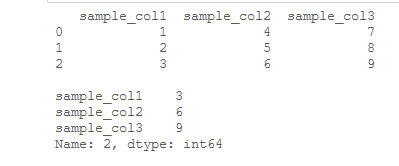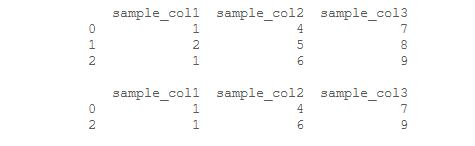# Get the specified row value of a given Pandas DataFrame

Pandas DataFrame is a two-dimensional size-mutable, potentially heterogeneous tabular data structure with labeled axes (rows and columns).

Now let’s see how to get the specified row value of a given DataFrame.

We shall be using loc[ ], iloc[ ], and [ ] for a data frame object to select rows and columns from our data frame.

1. iloc[ ] is used to select rows/ columns by their corresponding labels.
2. loc[ ] is used to select rows/columns by their indices.
3. [ ] is used to select columns by their respective names.

Method 1: Using iloc[ ].

Example: Suppose you have a pandas dataframe and you want to select a specific row given its index.

## Python3

 `# import pandas library ` `import` `pandas as pd ` ` `  `# Creating a dictionary ` `d ``=` `{``'sample_col1'``: [``1``, ``2``, ``3``], ` `     ``'sample_col2'``: [``4``, ``5``, ``6``],  ` `     ``'sample_col3'``: [``7``, ``8``, ``9``]}  ` ` `  `# Creating a Dataframe ` `df ``=` `pd.DataFrame(d)  ` ` `  `# show the dataframe  ` `print``(df)  ` ` `  `print``() ` ` `  `# Select Row No. 2 ` `print``(df.iloc[``2``])`

Output:Method 2: Using loc[ ].

Example: Suppose you want to select rows where the value of a given column is given.

## Python3

 `# import pandas library ` `import` `pandas as pd ` ` `  `# Creating a dictionary ` `d ``=` `{``'sample_col1'``: [``1``, ``2``, ``1``], ` `     ``'sample_col2'``: [``4``, ``5``, ``6``],  ` `     ``'sample_col3'``: [``7``, ``8``, ``9``]}  ` ` `  `# Creating a Dataframe ` `df ``=` `pd.DataFrame(d)  ` ` `  `# show the dataframe ` `print``(df)  ` ` `  `print``() ` ` `  `# Select rows where sample_col1 is 1 ` `print``(df.loc[df[``'sample_col1'``] ``=``=` `1``])`

Output:Method 3: Using [ ] and iloc[ ]

Example: Suppose you want only the values pertaining to specific columns of a specific row.

## Python3

 `# import pandas library ` `import` `pandas as pd ` ` `  `# Creating a dictionary ` `d ``=` `{``'sample_col1'``: [``1``, ``2``, ``1``], ` `     ``'sample_col2'``: [``4``, ``5``, ``6``],  ` `     ``'sample_col3'``: [``7``, ``8``, ``9``]}   ` ` `  `# Creating a Dataframe ` `df ``=` `pd.DataFrame(d)  ` ` `  `# show the dataframe ` `print``(df)  ` ` `  `print``() ` ` `  `# Display column 1 and 3 for row 2 ` `print``(df[[``'sample_col1'` `, ``'sample_col3'``]].iloc[``1``])`

Output:My Personal Notes arrow_drop_upEfficient Student pursuing a Computer Science degree with experience collaborating with teammates to create projects that solve real-world problems efficiently Well-versed in Data Structures and Algorithms, with a strong understanding of Mathematics, profound love for Neural Nets and Deep Learning

If you like GeeksforGeeks and would like to contribute, you can also write an article using contribute.geeksforgeeks.org or mail your article to contribute@geeksforgeeks.org. See your article appearing on the GeeksforGeeks main page and help other Geeks.

Please Improve this article if you find anything incorrect by clicking on the "Improve Article" button below.

Article Tags :

Be the First to upvote.

Please write to us at contribute@geeksforgeeks.org to report any issue with the above content.